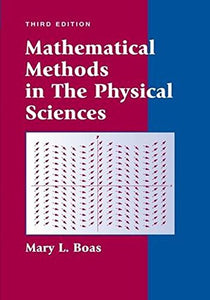Wiley

# Mathematical Methods In The Physical Sciences

• Publish Date: 2005-07-22
• Binding: Hardcover
• Author: Mary L. Boas

Regular price \$91.09 Sale price \$227.98

Attention: For textbook, access codes and supplements are not guaranteed with used items.

Now in its third edition,Mathematical Concepts in the Physical Sciences, 3rd Editionprovides a comprehensive introduction to the areas of mathematical physics. It combines all the essential math concepts into one compact, clearly written reference.

This book is intended for students who have had a two-semester or three-semester introductory calculus course. Its purpose is to help students develop, in a short time, a basic competence in each of the many areas of mathematics needed in advanced courses in physics, chemistry, and engineering. Students are given sufficient depth to gain a solid foundation (this is not a recipe book). At the same time, they are not overwhelmed with detailed proofs that are more appropriate for students of mathematics. The emphasis is on mathematical methods rather than applications, but students are given some idea of how the methods will be used along with some simple applications.